# Circle facts for kids

Kids Encyclopedia Facts

A circle is a round, two-dimensional shape. All points on the edge of the circle are at the same distance from the center.

The radius of a circle is a line from the centre of the circle to a point on the side. Mathematicians use the letter r for the length of a circle's radius. The centre of a circle is the point in the very middle. It is sometimes written as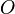$O$.

The diameter (meaning "all the way across") of a circle is a straight line that goes from one side to the opposite and right through the centre of the circle. Mathematicians use the letter d for the length of this line. The diameter of a circle is equal to twice its radius (d equals 2 times r):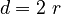$d = 2\ r$

The circumference (meaning "all the way around") of a circle is the line that goes around the centre of the circle. Mathematicians use the letter C for the length of this line.

The number π (written as the Greek letter pi) is a very useful number. It is the length of the circumference divided by the length of the diameter (π equals C divided by d). As a fraction the number π is equal to about 227 or 335/113 (which is closer) and as a number it is about 3.1415926535.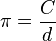$\pi = \frac{C}{d}$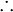$\therefore$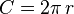$C = 2\pi \, r$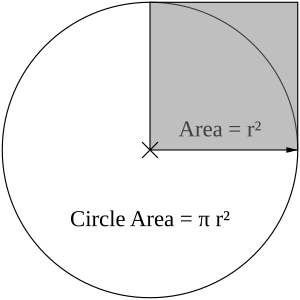The area of the circle is equal to π times the area of the gray square.

The area, A, inside a circle is equal to the radius multiplied by itself, then multiplied by π (A equals π times r times r).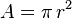$A = \pi \, r^2$

## Calculating π

π can be measured by drawing a large circle, then measuring its diameter (d) and circumference (C). This is because the circumference of a circle is always π times its diameter.$\pi = \frac{C}{d}$

π can also be calculated by only using mathematical methods. Most methods used for calculating the value of π have desirable mathematical properties. However, they are hard to understand without knowing trigonometry and calculus. However, some methods are quite simple, such as this form of the Gregory-Leibniz series: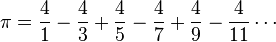$\pi = \frac{4}{1}-\frac{4}{3}+\frac{4}{5}-\frac{4}{7}+\frac{4}{9}-\frac{4}{11}\cdots$.

While that series is easy to write and calculate, it is not easy to see why it equals π. A much easier way to approach is to draw an imaginary circle of radius r centered at the origin. Then any point (x,y) whose distance d from the origin is less than r, calculated by the Pythagorean theorem, will be inside the circle: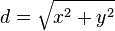$d = \sqrt{x^2 + y^2}$

Finding a set of points inside the circle allows the circle's area A to be estimated, for example, by using integer coordinates for a big r. Since the area A of a circle is π times the radius squared, π can be approximated by using the following formula: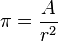$\pi = \frac{A}{r^2}$

## Images for kidsCircle Facts for Kids. Kiddle Encyclopedia.